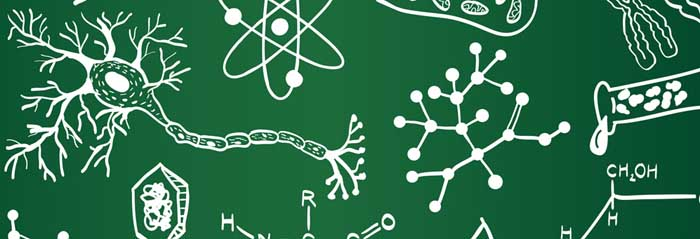Engg. Entrance Exams

PULEET SyllabusGeneral Engineering Syllabus:

Basic Electrical Engineering:

1. DC circuits
2. Single Phase AC Fundamentals
3. Three Phase AC Fundamentals
4. Magnetic Circuit
5. Transformers
6. Electric Machines

Basic Electronics:

1. Semiconductor Diode
2. Bipolar Junction Transistor
3. Field Effect Transistor
4. Operational Amplifiers
5. Oscillators
6. Boolean Algebra and Logic Gates
7. Flip Flops
8. Test and Measuring Instruments
9. Communication

Programming Fundamentals:

1. Introduction
2. Basic Constructs of C
3. Program Control Flow
4. Arrays & Functions
5. Structures
6. Input and Output
7. Introduction to Object Oriented Programming

Fundamentals of Mechanical Engineering:

1. Laws Of Thermodynamics
2. Steam and Its Formation
3. Power Cycles
4. Kinematics Of Fluid Flow
5. Fluid Dynamics
6. Flow Measurement
7. Simple Stress and Strains
8. Bending moment (B.M.) and Shear force (S.F.)
9. Bending and Torsion

Physics:

Mechanics:

Linear kinematics and its equations of motion, projectile motion, circular motion. Newton’s laws of motion, principle of conservation of momentum applications to linear and planar motion, concept of friction and its laws, motion on smooth and rough inclined planes, simple and complex Atwood’s machines.

Concept of work, energy and power, work-energy principle, principle of conservation of energy.

Rotational motion, equations of rotational kinematics, moment of inertia and radius of gyration of a rotating body; torque and angular momentum; work, power and energy in rotational motion, conservation of angular momentum. Simple harmonic motion and its characteristics, energetics of simple harmonic motion, idea of damped and forced oscillations, resonance and its applications.

Wave motion and its characteristics, theory of sound propagation, velocity of sound and factors influencing the velocity of sound, Doppler effect in sound, superposition of sound in space (stationary waves) and time (beats), vibrations of air columns and stretched strings.

Optics:

Geometrical optics, reflection and refraction of light, reflections by spherical mirrors, refraction through lenses, spherical and chromatic aberrations, dispersion and deviation of light through prism, optical microscopes and telescopes.

Wave nature of light; interference, Young’s double slit experiment, Lloyd’s mirror and Fresenel’s biprism techniques for producing interference pattern, interference through thin film, colouring of thin films; diffraction of light through a single slit, Rayleigh’s criteria of resolution, resolving power of optical instruments ; concept of polarization, methods of producing polarized light, analysis of polarized light, Doppler effect in light.

Laser, its principle, characteristics and applications.

Thermodynamic and Thermochemistry:

Thermometry, idea of specific heat and heat capacity, latent heats of fusion and vaporization, variation of specific heats of solids, liquids and gases with temperature, concept of degree of freedom, law of equipartition of energy. Modes of heat transfer (conduction, convection and radiation); linear, surface and volume expansion of matter on heating.

Electrodynamics:

Electric field and electric potential, electric dipole and its field, Gauss’ law and its applications; concept of capacitance, energy stored in a capacitor, effect of introducing dielectric and conducting slabs between plates of a capacitor, dielectric constant of material.

Current electricity, Kirchoff’s laws and applications, thermal and chemical effects of current, slide wire bridge, potentiometer, ammeter and voltmeter.

Magnetic effects of current, Biot-Savert law and its applications, Lorentz force, moving coil galvanometers ; laws of electromagnetic induction, eddy currents and its applications, self and mutual inductance.

Modern Physics:

Black body radiation distribution, photoelectric effect, idea of x-ray production, wave-matter duality and de-Broglie waves, positionmomentum uncertainty principle.

Crystalline and amorphous solids, basic idea of crystal structures (simple cubic, body- centered cubic, face-centered cubic) and their characteristics, close packing morphologies, Schottkey and Frenkel defects in crystals. Rutherford scattering experiment, Bohr’s model and hydrogen spectrum. Nucleus and its properties (mass, size, binding energy, magnetic and quadruple moments), nuclear forces and its properties, phenomenon of radioactivity and its laws, modes of radioactive decays (α, β and γ), nuclear fission and fusion.

Maths:

1. Algebra
2. Matrices
3. Trigonometry
4. Coordinate Geometry
5. Differential Calculus of Functions of one variable
6. Differential Calculus of Functions of two variables
7. Ordinary Differential Equations
8. Integral Calculus
9. Vector Differential Calculus
10. Vector Integral Calculus

Environment Education:

1. Ecology and Ecosystems
2. Biodiversity and its conservation
3. Natural Resources
4. Environmental Pollution